A step-by-step complete beginner’s guide to building your first Neural Network in a couple lines of code like a Deep Learning pro!

Writing your first Neural Network can be done with merely a couple lines of code! In this post, we will be exploring how to use a package called Keras to build our first neural network to predict if house prices are above or below median value. In particular, we will go through the full Deep Learning pipeline, from:

• Exploring and Processing the Data
• Building and Training our Neural Network
• Visualizing Loss and Accuracy
• Adding Regularization to our Neural Network

In just 20 to 30 minutes, you will have coded your own neural network just as a Deep Learning practitioner would have!

Pre-requisites:

This post assumes you’ve got Jupyter notebook set up with an environment that has the packages keras, tensorflow, pandas, scikit-learn and matplotlib installed. If you have not done so, please follow the instructions in the tutorial below:

This is a Coding Companion to Intuitive Deep Learning Part 1. As such, we assume that you have some intuitive understanding of neural networks and how they work, including some of the nitty-gritty details, such as what overfitting is and the strategies to address them. If you need a refresher, please read these intuitive introductions:

Resources you need:

The dataset we will use today is adapted from Zillow’s Home Value Prediction Kaggle competition data. We’ve reduced the number of input features and changed the task into predicting whether the house price is above or below median value. Please visit the below link to download the modified dataset below and place it in the same directory as your notebook. The download icon should be on the top right.

Optionally, you may also download an annotated Jupyter notebook which has all the code covered in this post: Jupyter Notebook.

Note that to download this notebook from Github, you have to go to the front page and download ZIP to download all the files:

And now, let’s begin!

Exploring and Processing the Data

Before we code any ML algorithm, the first thing we need to do is to put our data in a format that the algorithm will want. In particular, we need to:

• Read in the CSV (comma separated values) file and convert them to arrays. Arrays are a data format that our algorithm can process.
• Split our dataset into the input features (which we call x) and the label (which we call y).
• Scale the data (we call this normalization) so that the input features have similar orders of magnitude.
• Split our dataset into the training set, the validation set and the test set. If you need a refresher on why we need these three datasets, please refer to Intuitive Deep Learning Part 1b.

So let’s begin! From the Getting Started with Python for Deep Learning and Data Science tutorial, you should have downloaded the package pandas to your environment. We will need to tell our notebook that we will use that package by importing it. Type the following code and press Alt-Enter on your keyboard:

import pandas as pd

This just means that if I want to refer to code in the package ‘pandas’, I’ll refer to it with the name pd. We then read in the CSV file by running this line of code:

This line of code means that we will read the csv file ‘housepricedata.csv’ (which should be in the same directory as your notebook) and store it in the variable ‘df’. If we want to find out what is in df, simply type df into the grey box and click Alt-Enter:

df

Your notebook should look something like this:

Here, you can explore the data a little. We have our input features in the first ten columns:

• Lot Area (in sq ft)
• Overall Quality (scale from 1 to 10)
• Overall Condition (scale from 1 to 10)
• Total Basement Area (in sq ft)
• Number of Full Bathrooms
• Number of Half Bathrooms
• Number of Bedrooms above ground
• Total Number of Rooms above ground
• Number of Fireplaces
• Garage Area (in sq ft)

In our last column, we have the feature that we would like to predict:

• Is the house price above the median or not? (1 for yes and 0 for no)

Now that we’ve seen what our data looks like, we want to convert it into arrays for our machine to process:

dataset = df.values

To convert our dataframe into an array, we just store the values of df (by accessing df.values) into the variable ‘dataset’. To see what is inside this variable ‘dataset’, simply type ‘dataset’ into a grey box on your notebook and run the cell (Alt-Enter):

dataset

As you can see, it is all stored in an array now: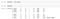Converting our dataframe into an array

We now split our dataset into input features (X) and the feature we wish to predict (Y). To do that split, we simply assign the first 10 columns of our array to a variable called X and the last column of our array to a variable called Y. The code to do the first assignment is this:

X = dataset[:,0:10]

This might look a bit weird, but let me explain what’s inside the square brackets. Everything before the comma refers to the rows of the array and everything after the comma refers to the columns of the arrays.

Since we’re not splitting up the rows, we put ‘:’ before the comma. This means to take all the rows in dataset and put it in X.

We want to extract out the first 10 columns, and so the ‘0:10’ after the comma means take columns 0 to 9 and put it in X (we don’t include column 10). Our columns start from index 0, so the first 10 columns are really columns 0 to 9.

We then assign the last column of our array to Y:

Y = dataset[:,10]

Ok, now we’ve split our dataset into input features (X) and the label of what we want to predict (Y).

The next step in our processing is to make sure that the scale of the input features are similar. Right now, features such as lot area are in the order of the thousands, a score for overall quality is ranged from 1 to 10, and the number of fireplaces tend to be 0, 1 or 2.

This makes it difficult for the initialization of the neural network, which causes some practical problems. One way to scale the data is to use an existing package from scikit-learn (that we’ve installed in the Getting Started post).

We first have to import the code that we want to use:

from sklearn import preprocessing

This says I want to use the code in ‘preprocessing’ within the sklearn package. Then, we use a function called the min-max scaler, which scales the dataset so that all the input features lie between 0 and 1 inclusive:

min_max_scaler = preprocessing.MinMaxScaler()
X_scale = min_max_scaler.fit_transform(X)

Note that we chose 0 and 1 intentionally to aid the training of our neural network. We won’t go through the theory behind this. Now, our scaled dataset is stored in the array ‘X_scale’. If you wish to see what ‘X_scale’ looks like, simply run the cell:

X_scale

Your Jupyter notebook should now look a bit like this:

Now, we are down to our last step in processing the data, which is to split our dataset into a training set, a validation set and a test set.

We will use the code from scikit-learn called ‘train_test_split’, which as the name suggests, split our dataset into a training set and a test set. We first import the code we need:

from sklearn.model_selection import train_test_split

Then, split your dataset like this:

X_train, X_val_and_test, Y_train, Y_val_and_test = train_test_split(X_scale, Y, test_size=0.3)

This tells scikit-learn that your val_and_test size will be 30% of the overall dataset. The code will store the split data into the first four variables on the left of the equal sign as the variable names suggest.

Unfortunately, this function only helps us split our dataset into two. Since we want a separate validation set and test set, we can use the same function to do the split again on val_and_test:

X_val, X_test, Y_val, Y_test = train_test_split(X_val_and_test, Y_val_and_test, test_size=0.5)

The code above will split the val_and_test size equally to the validation set and the test set.

In summary, we now have a total of six variables for our datasets we will use:

• X_train (10 input features, 70% of full dataset)
• X_val (10 input features, 15% of full dataset)
• X_test (10 input features, 15% of full dataset)
• Y_train (1 label, 70% of full dataset)
• Y_val (1 label, 15% of full dataset)
• Y_test (1 label, 15% of full dataset)

If you want to see how the shapes of the arrays are for each of them (i.e. what dimensions they are), simply run

print(X_train.shape, X_val.shape, X_test.shape, Y_train.shape, Y_val.shape, Y_test.shape)

This is how your Jupyter notebook should look like:

As you can see, the training set has 1022 data points while the validation and test set has 219 data points each. The X variables have 10 input features, while the Y variables only has one feature to predict.

And now, our data is finally ready! Phew!

Summary: In processing the data, we’ve:

• Read in the CSV (comma separated values) file and convert them to arrays.
• Split our dataset into the input features and the label.
• Scale the data so that the input features have similar orders of magnitude.
• Split our dataset into the training set, the validation set and the test set.

Building and Training our First Neural Network

In Intuitive Deep Learning Part 1a, we said that Machine Learning consists of two steps. The first step is to specify a template (an architecture) and the second step is to find the best numbers from the data to fill in that template. Our code from here on will also follow these two steps.

First Step: Setting up the Architecture

The first thing we have to do is to set up the architecture. Let’s first think about what kind of neural network architecture we want. Suppose we want this neural network: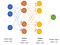Neural network architecture that we will use for our problem

In words, we want to have these layers:

• Hidden layer 1: 32 neurons, ReLU activation
• Hidden layer 2: 32 neurons, ReLU activation
• Output Layer: 1 neuron, Sigmoid activation

Now, we need to describe this architecture to Keras. We will be using the Sequential model, which means that we merely need to describe the layers above in sequence.

First, let’s import the necessary code from Keras:

from keras.models import Sequential
from keras.layers import Dense

Then, we specify that in our Keras sequential model like this:

model = Sequential([
Dense(32, activation='relu', input_shape=(10,)),
Dense(32, activation='relu'),
Dense(1, activation='sigmoid'),
])

And just like that, the code snippet above has defined our architecture! The code above can be interpreted like this:

model = Sequential([ ... ])

This says that we will store our model in the variable ‘model’, and we’ll describe it sequentially (layer by layer) in between the square brackets.

Dense(32, activation='relu', input_shape=(10,)),

We have our first layer as a dense layer with 32 neurons, ReLU activation and the input shape is 10 since we have 10 input features. Note that ‘Dense’ refers to a fully-connected layer, which is what we will be using.

Dense(32, activation='relu'),

Our second layer is also a dense layer with 32 neurons, ReLU activation. Note that we do not have to describe the input shape since Keras can infer from the output of our first layer.

Dense(1, activation='sigmoid'),

Our third layer is a dense layer with 1 neuron, sigmoid activation.

And just like that, we have written our model architecture (template) in code!

Second Step: Filling in the best numbers

Now that we’ve got our architecture specified, we need to find the best numbers for it. Before we start our training, we have to configure the model by

• Telling it which algorithm you want to use to do the optimization
• Telling it what loss function to use
• Telling it what other metrics you want to track apart from the loss function

Configuring the model with these settings requires us to call the function model.compile, like this:

model.compile(optimizer='sgd',
loss='binary_crossentropy',
metrics=['accuracy'])

We put the following settings inside the brackets after model.compile:

optimizer='sgd'

‘sgd’ refers to stochastic gradient descent (over here, it refers to mini-batch gradient descent), which we’ve seen in Intuitive Deep Learning Part 1b.

loss='binary_crossentropy'

The loss function for outputs that take the values 1 or 0 is called binary cross entropy.

metrics=['accuracy']

Lastly, we want to track accuracy on top of the loss function. Now once we’ve run that cell, we are ready to train!

Training on the data is pretty straightforward and requires us to write one line of code:

hist = model.fit(X_train, Y_train,
batch_size=32, epochs=100,
validation_data=(X_val, Y_val))

The function is called ‘fit’ as we are fitting the parameters to the data. We have to specify what data we are training on, which is X_train and Y_train. Then, we specify the size of our mini-batch and how long we want to train it for (epochs). Lastly, we specify what our validation data is so that the model will tell us how we are doing on the validation data at each point. This function will output a history, which we save under the variable hist. We’ll use this variable a little later when we get to visualization.

Now, run the cell and watch it train! Your Jupyter notebook should look like this:

You can now see that the model is training! By looking at the numbers, you should be able to see the loss decrease and the accuracy increase over time. At this point, you can experiment with the hyper-parameters and neural network architecture. Run the cells again to see how your training has changed when you’ve tweaked your hyperparameters.

Once you’re happy with your final model, we can evaluate it on the test set. To find the accuracy on our test set, we run this code snippet:

model.evaluate(X_test, Y_test)

The reason why we have the index 1 after the model.evaluate function is because the function returns the loss as the first element and the accuracy as the second element. To only output the accuracy, simply access the second element (which is indexed by 1, since the first element starts its indexing from 0).

Due to the randomness in how we have split the dataset as well as the initialization of the weights, the numbers and graph will differ slightly each time we run our notebook. Nevertheless, you should get a test accuracy anywhere between 80% to 95% if you’ve followed the architecture I specified above!

And there you have it, you’ve coded up your very first neural network and trained it! Congratulations!

Summary: Coding up our first neural network required only a few lines of code:

• We specify the architecture with the Keras Sequential model.
• We specify some of our settings (optimizer, loss function, metrics to track) with model.compile
• We train our model (find the best parameters for our architecture) with the training data with model.fit
• We evaluate our model on the test set with model.evaluate

Visualizing Loss and Accuracy

In Intuitive Deep Learning Part 1b, we talked about overfitting and some regularization techniques. How do we know if our model is currently overfitting?

What we might want to do is to plot the training loss and the val loss over the number of epochs passed. To display some nice graphs, we will use the package matplotlib. As usual, we have to import the code we wish to use:

import matplotlib.pyplot as plt

Then, we want to visualize the training loss and the validation loss. To do so, run this snippet of code:

plt.plot(hist.history['loss'])
plt.plot(hist.history['val_loss'])
plt.title('Model loss')
plt.ylabel('Loss')
plt.xlabel('Epoch')
plt.legend(['Train', 'Val'], loc='upper right')
plt.show()

We’ll explain each line of the above code snippet. The first two lines says that we want to plot the loss and the val_loss. The third line specifies the title of this graph, “Model Loss”. The fourth and fifth line tells us what the y and x axis should be labelled respectively. The sixth line includes a legend for our graph, and the location of the legend will be in the upper right. And the seventh line tells Jupyter notebook to display the graph.

Your Jupyter notebook should look something like this: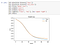A graph of model loss that you should see in your Jupyter notebook

We can do the same to plot our training accuracy and validation accuracy with the code below:

plt.plot(hist.history['acc'])
plt.plot(hist.history['val_acc'])
plt.title('Model accuracy')
plt.ylabel('Accuracy')
plt.xlabel('Epoch')
plt.legend(['Train', 'Val'], loc='lower right')
plt.show()

You should get a graph that looks a bit like this: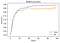Plot of model accuracy for training and validation set

Since the improvements in our model to the training set looks somewhat matched up with improvements to the validation set, it doesn’t seem like overfitting is a huge problem in our model.

Summary: We use matplotlib to visualize the training and validation loss / accuracy over time to see if there’s overfitting in our model.

Adding Regularization to our Neural Network

For the sake of introducing regularization to our neural network, let’s formulate with a neural network that will badly overfit on our training set. We’ll call this Model 2.

model_2 = Sequential([
Dense(1000, activation='relu', input_shape=(10,)),
Dense(1000, activation='relu'),
Dense(1000, activation='relu'),
Dense(1000, activation='relu'),
Dense(1, activation='sigmoid'),
])
loss='binary_crossentropy',
metrics=['accuracy'])
hist_2 = model_2.fit(X_train, Y_train,
batch_size=32, epochs=100,
validation_data=(X_val, Y_val))

Here, we’ve made a much larger model and we’ve use the Adam optimizer. Adam is one of the most common optimizers we use, which adds some tweaks to stochastic gradient descent such that it reaches the lower loss function faster. If we run this code and plot the loss graphs for hist_2 using the code below (note that the code is the same except that we use ‘hist_2’ instead of ‘hist’):

plt.plot(hist_2.history['loss'])
plt.plot(hist_2.history['val_loss'])
plt.title('Model loss')
plt.ylabel('Loss')
plt.xlabel('Epoch')
plt.legend(['Train', 'Val'], loc='upper right')
plt.show()

We get a plot like this:

This is a clear sign of over-fitting. The training loss is decreasing, but the validation loss is way above the training loss and increasing (past the inflexion point of Epoch 20). If we plot accuracy using the code below:

plt.plot(hist_2.history['acc'])
plt.plot(hist_2.history['val_acc'])
plt.title('Model accuracy')
plt.ylabel('Accuracy')
plt.xlabel('Epoch')
plt.legend(['Train', 'Val'], loc='lower right')
plt.show()

We can see a clearer divergence between train and validation accuracy as well: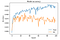Training and validation accuracy for our overfitting model

Now, let’s try out some of our strategies to reduce over-fitting (apart from changing our architecture back to our first model). Remember from Intuitive Deep Learning Part 1b that we introduced three strategies to reduce over-fitting.

Of the three, we’ll incorporate L2 regularization and dropout here. The reason we don’t add early stopping here is because after we’ve used the first two strategies, the validation loss doesn’t take the U-shape we see above and so early stopping will not be as effective.

First, let’s import the code that we need for L2 regularization and dropout:

from keras.layers import Dropout
from keras import regularizers

We then specify our third model like this:

model_3 = Sequential([
Dense(1000, activation='relu', kernel_regularizer=regularizers.l2(0.01), input_shape=(10,)),
Dropout(0.3),
Dense(1000, activation='relu', kernel_regularizer=regularizers.l2(0.01)),
Dropout(0.3),
Dense(1000, activation='relu', kernel_regularizer=regularizers.l2(0.01)),
Dropout(0.3),
Dense(1000, activation='relu', kernel_regularizer=regularizers.l2(0.01)),
Dropout(0.3),
Dense(1, activation='sigmoid', kernel_regularizer=regularizers.l2(0.01)),
])

Can you spot the differences between Model 3 and Model 2? There are two main differences:

Difference 1: To add L2 regularization, notice that we’ve added a bit of extra code in each of our dense layers like this:

kernel_regularizer=regularizers.l2(0.01)

This tells Keras to include the squared values of those parameters in our overall loss function, and weight them by 0.01 in the loss function.

Difference 2: To add Dropout, we added a new layer like this:

Dropout(0.3),

This means that the neurons in the previous layer has a probability of 0.3 in dropping out during training. Let’s compile it and run it with the same parameters as our Model 2 (the overfitting one):

loss='binary_crossentropy',
metrics=['accuracy'])
hist_3 = model_3.fit(X_train, Y_train,
batch_size=32, epochs=100,
validation_data=(X_val, Y_val))

And now, let’s plot the loss and accuracy graphs. You’ll notice that the loss is a lot higher at the start, and that’s because we’ve changed our loss function. To plot such that the window is zoomed in between 0 and 1.2 for the loss, we add an additional line of code (plt.ylim) when plotting:

plt.plot(hist_3.history['loss'])
plt.plot(hist_3.history['val_loss'])
plt.title('Model loss')
plt.ylabel('Loss')
plt.xlabel('Epoch')
plt.legend(['Train', 'Val'], loc='upper right')
plt.ylim(top=1.2, bottom=0)
plt.show()

We’ll get a loss graph that looks like this:

You can see that the validation loss much more closely matches our training loss. Let’s plot the accuracy with similar code snippet:

plt.plot(hist_3.history['acc'])
plt.plot(hist_3.history['val_acc'])
plt.title('Model accuracy')
plt.ylabel('Accuracy')
plt.xlabel('Epoch')
plt.legend(['Train', 'Val'], loc='lower right')
plt.show()

And we will get a plot like this:

Compared to our model in Model 2, we’ve reduced overfitting substantially! And that’s how we apply our regularization techniques to reduce overfitting to the training set.

Summary: To deal with overfitting, we can code in the following strategies into our model each with about one line of code:

• L2 Regularization
• Dropout

If we visualize the training / validation loss and accuracy, we can see that these additions have helped deal with overfitting!

Consolidated Summary: In this post, we’ve written Python code to:

• Explore and Process the Data
• Build and Train our Neural Network
• Visualize Loss and Accuracy
• Add Regularization to our Neural Network

We’ve been through a lot, but we haven’t written too many lines of code! Building and Training our Neural Network has only taken about 4 to 5 lines of code, and experimenting with different model architectures is just a simple matter of swapping in different layers or changing different hyperparameters. Keras has indeed made it a lot easier to build our neural networks, and we’ll continue to use it for more advanced applications in Computer Vision and Natural Language Processing.

What’s Next: In our next Coding Companion Part 2, we will explore how to code up our own Convolutional Neural Networks (CNNs) to do image recognition!

Be sure to first get an intuitive understanding of CNNs here: Intuitive Deep Learning Part 2: CNNs for Computer Vision

Written by

Intuitive Deep Learning

We explain cutting-edge deep learning concepts in an intuitive way! Non-technical beginners, students of deep learning and industry professionals can get a gentle introduction into technical concepts without too much math and code to bog you down!

Welcome to a place where words matter. On Medium, smart voices and original ideas take center stage - with no ads in sight. Watch
Follow all the topics you care about, and we’ll deliver the best stories for you to your homepage and inbox. Explore
Get unlimited access to the best stories on Medium — and support writers while you’re at it. Just \$5/month. Upgrade